# Institut für Thermodynamik der Luft- und Raumfahrt - Universität StuttgartMechanical Engineering - The University of Texas at Austin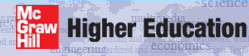# construction of the dataset

The contents of the laminar pipe flow benchmark dataset, s30.dat.txt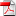, is described in four data blocks:

## Data Block ONE - Geometry, PDE's, Models, Units, and Fluid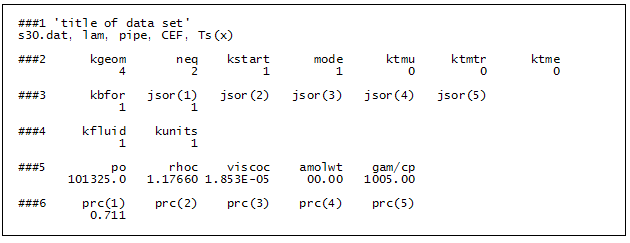### ###1

'title of dataset'
s30.dat, lam, pipe, CEF, Ts(x)

• title - to appear in all output files

This is a descriptive user-written alphanumeric statement of 80 characters maximum

### ###2

kgeom  neq  kstart  mode  ktmu  ktmtr  ktme
4  2  1  1  0  0  0

• kgeom - flag for the type of geometry

=4   is the circular pipe geometry.

Note that for axisymmetric geometries TEXSTAN computes the flow on a per unit radian basis. Integration of the heat flux to determine total heat transfer will require multiplying the result by two pi.

• neq - flag for the number of transport equations to be solved

=2   means TEXSTAN will solve the momentum transport equation and one other transport equation. The problem statement specifies investigation of friction and heat transfer characteristics of combined entry flow in a pipe. Therefore two equations need to be solved (momentum and energy).

The pipe problem could be considered either a pure momentum problem (friction) or it can be considered a combined momentum and heat transfer problem. The flag that will define the "other transport equation" is the variable jsor. For the pipe problem the user will choose jsor =1 and that will tell TEXSTAN that the second transport equation is a thermal energy equation.

Note that if the pipe flow solution for momentum is all that is required, setting neq =1 will eliminate solution to the thermal energy equation.

• kstart - flag to specify the type of initial profiles and whether they will be TEXSTAN-generated or input from tables

=1   instructs TEXSTAN to compute flat initial profiles for velocity and temperature to start the calculations, in this case at the entrance to the pipe.

• mode - flag to tell TEXSTAN whether to compute turbulent viscosity at the start of the calculations

=1   means the flow will start laminar, which is correct for the pipe flow problem. Note - TEXSTAN will compute laminar pipe flow at any Reynolds number as long as mode is set =1.

• ktmu - the flag to tell TEXSTAN what to use for a momentum equation turbulence model

=0    This flag value means the flow will remain laminar. For internal flows, ktmu =0 is required if mode =1 because transition is not permitted.

• ktmtr - the flag to tell TEXSTAN what to use for a transition turbulence model

=0   for all internal flows - this turbulent transition variable is not used - the flow state is determined completely by the variable mode (if it is set =2, then a ktmu must be specified). Note TEXSTAN input format does not permit a blank for a zero value.

• ktme - flag to tell TEXSTAN what to use for an energy equation turbulence model

=0   This value is consistent with ktmu =0 even though heat transfer is being considered.

### ###3

kbfor  jsor(1)  jsor(2)  jsor(3)  jsor(4)  jsor(5)
1  1

• kbfor - the flag to tell TEXSTAN what body forces to include in the momentum equation source term

=1   means only the pressure gradient, dp/dx, will be calculated as a momentum source term. This value is used for most flow problems unless free convective body forces are also being considered.

• jsor(j) - the flag to tell TEXSTAN what each diffusion equation will be and which set of source terms to include when formulating the general source for this diffusion equation

jsor(j=1)=1    The problem statement tells us that heat transfer is being considered, so the jsor(j=1) value will have to be in the range between 1-8. In this range TEXSTAN knows this diffusion equation is the energy equation, and by setting the value =1 we tell TEXSTAN not to compute energy source terms for viscous dissipation or work against body forces.

jsor(j=2) - jsor(j=5) flags will be left blank because neq=2 for this pipe problem, meaning only (neq-1)=1 diffusion equations will be solved and TEXSTAN will not need to read the other array values.

### ###4

kfluid  kunits
1  1

• kfluid - the flag to tell TEXSTAN whether to use constant or variable properties

=1   is the choice for constant properties, a requirement for this pipe flow problem.

• kunits - the flag to tell TEXSTAN whether to use SI (metric) units or U.S. Customary (English/British) units for all physical variables

=1   is the choice to use SI units.

### ###5

po  rhoc  viscoc  amolwt  gam/cp
1013325.0  1.17660  1.853E-05  00.00  1005.00

• po - the fluid pressure at the start of computations, x = xstart

=101325.0   in units of N/m2 or Pa - (atmospheric pressure) - the fluid pressure will be interpreted as static pressure rather than total pressure for all internal flows, regardless of whether the properties are constant or variable.

• rhoc - the fluid mass density

=1.17660    in units of kg/m3 - (air at about 325 K and one atmosphere).

• viscoc - the fluid dynamic viscosity

=1.853E-05    in units of (N-s)/m2 or (Pa-s) - (air at about 325 K).

• amolwt - the fluid molecular weight

=00.00    variable not required for constant property calculations - TEXSTAN input format does not permit a blank for a zero value.

• gam/cp - the ratio of the fluid specific heats (or) the fluid specific heat

=1005.00   in units of J/(kg-K) - (specific heat at constant pressure for air at about 325 K) - the variable is interpreted as the fluid specific heat, rather than gamma, the ratio of specific heats, because of the constant properties assumption, kfluid =1.

### ###6

prc(1)  prc(2)  prc(3)  prc(4)  prc(5)
0.700

• prc(j) - generalized transport equation C variable for each diffusion equation

prc(j=1)=0.700   TEXSTAN interprets prc(j=1) as a Prandtl number (in this problem Pr for air at about 325K) because jsor(j=1) was set =1 (meaning the first diffusion equation is an energy equation).

prc(j=2) - prc(j=5) flags will be left blank because neq=2 for this pipe flow problem, meaning only (neq-1)=1 diffusion equations will be solved and TEXSTAN will not need to read the other array values.

## Data Block TWO - Boundary Conditions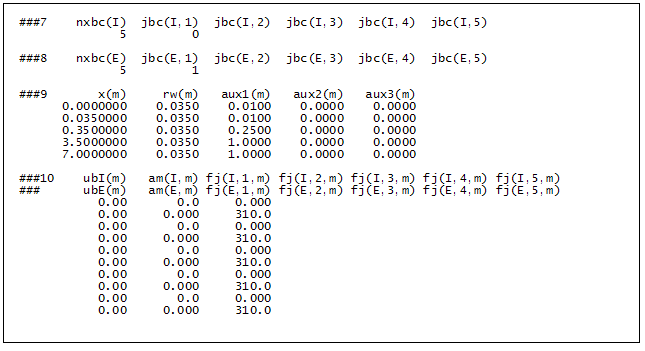### ###7 (and) ###8

nxbc(I)  jbc(I,1)  jbc(I,2)  jbc(I,3)  jbc(I,4)  jbc(I,5)
5  0
nxbc(E)  jbc(E,1)  jbc(E,2)  jbc(E,3)  jbc(E,4)  jbc(E,5)
5  1

• nxbc(I) - number of boundary condition locations, I-surface

nxbc(I)=5    indicates there will be a total of five x-locations where boundary conditions are specified. The interior 3 locations are NOT arbitrary locations. They are determined using the internal flows: integration control section of this website to specify integration stepsize change locations. Note that you can define other x-locations for obtaining velocity and temperature profiles when the k10 variable is reset =10 or =11.

• nxbc(E)=nxbc(I) - number of boundary condition locations, E-surface

nxbc(E)=5    This value must be identical to nxbc(I)

• jbc(I,j) - the flag to tell TEXSTAN type of boundary condition at the I-surface (Dirichlet or level, Neumann or flux, or symmetry line) for each diffusion equation

jbc(I,j=1)=0   tells TEXSTAN that the boundary condition along the I-surface for the first diffusion equation (the energy equation) will be mathematically a special Neumann or flux type of zero gradient (symmetry boundary condition).

jbc(I,j=2) - jbc(I,j=5) flags will be left blank because neq=2 for this pipe problem, meaning only (neq-1)=1 diffusion equations will be solved and TEXSTAN will not need to read the other values in the array.

• jbc(E,j) - the flag to tell TEXSTAN type of boundary condition at the E-surface (Dirichlet or level, Neumann or flux, or symmetry line) for each diffusion equation

jbc(E,j=1)=1   tells TEXSTAN that the boundary condition along the E-surface for the first diffusion equation (the energy equation) will be mathematically a Dirichlet or level type, which translates into a surface temperature distribution along the E-surface (if it had been =2, it would have been mathematically a Neumann or flux type, which would imply a surface heat flux distribution along the E-surface).

jbc(E,j=2) - jbc(E,j=5) flags will be left blank because neq=2 for this pipe problem, meaning only (neq-1)=1 diffusion equations will be solved and TEXSTAN will not need to read the other values in the array.

### ###9

x(m)  rw(m)  aux1(m)  aux2(m)  aux3(m)
0.0000000  0.0350  0.0100  0.0000  0.0000
0.0350000  0.0350  0.0100  0.0000  0.0000
0.3500000  0.0350  0.2500  0.0000  0.0000
3.5000000  0.0350  1.0000  0.0000  0.0000
7.0000000  0.0350  1.0000  0.0000  0.0000

• x(m) - the boundary condition x-location array

As seen in the x(m) array table, there are five points, including x(1)=0.0 and x(5)=7.0 (the pipe length in meters for this problem). The x-locations are determined using the internal flows: integration control section of this website to specify integration stepsize change locations. Note that you can define other x-locations for obtaining velocity and temperature profiles when the k10 variable is reset =10 or =11.

• rw(m) - the transverse curvature array that contributes to defining a given geometry

As seen in the rw(m) array table, these values are set =0.035 (the pipe radius in meters for this problem).

• aux1(m) - an auxiliary array that is used to provide additional input data to TEXSTAN

The aux1(m) array table values are stepsize values determined using the internal flows: integration control section of this website. The array is used in place of deltax whenever the flag kdx is set =1.

• aux2(m) - an auxiliary array that is used to provide additional input data to TEXSTAN

As seen in the aux2(m) array table, this variable is not required for the Blasius problem, but it must be filled with zero values because it is part of the structured data input for TEXSTAN.

• aux3(m) - an auxiliary array that is used to provide additional input data to TEXSTAN

As seen in the aux3(m) array table, this variable is not required for the Blasius problem, but it must be filled with zero values because it is part of the structured data input for TEXSTAN.

### ###10

ubI(m)  am(I,m)  fj(I,1,m)  fj(I,2,m)  fj(I,3,m)  fj(I,4,m)  fj(I,5,m)
ubE(m)  am(E,m)  fj(E,1,m)  fj(E,2,m)  fj(E,3,m)  fj(E,4,m)  fj(E,5,m)
0.00  0.0  0.000
0.00  0.000  310.0
0.00  0.0  0.000
0.00  0.000  310.0
0.00  0.0  0.000
0.00  0.000  310.0
0.00  0.0  0.000
0.00  0.000  310.0
0.00  0.0  0.000
0.00  0.000  310.0

• ubI(m) - the momentum flow-direction velocity boundary condition array at the I-surface

The ubI(m) array for internal flow geometries kgeom=4,5 is a symmetry line velocity boundary condition at the pipe or channel centerline, and all values are set =0.00.

• ubE(m) - the momentum flow-direction velocity boundary condition array at the E-surface

The ubE(m) array for all internal flow geometries is the no-slip velocity boundary condition at the solid surface, and all values are set =0.00.

• am(I,m) - the momentum cross-stream mass flux boundary condition array at the I-surface

The am(I,m) array for all internal flow geometries is set =0.0. The (cross-stream or y-directed mass flux) is not used for internal flows.

• am(E,m) - the momentum cross-stream ( or y-directed) mass flux boundary condition array at the E-surface

The am(E,m) array for all internal flow geometries is set =0.0. The (cross-stream or y-directed mass flux) is not used for internal flows.

• fj(I,j,m) - the diffusion equation boundary condition array at the I-surface for each j at each x(m) location

array for internal flow geometries kgeom=4,5 is a symmetry line diffusion-equation boundary condition at the pipe or channel centerline, and all values are set =0.00, regardless of the type of diffusion equation.

fj(I,j=2,m) - fj(I,j=5,m) variables will be left blank because neq=2 for this pipe flow problem, meaning only (neq-1)=1 diffusion equations will be solved and TEXSTAN will not need to read fj(I,j=2,m) - fj(I,j=5,m).

• fj(E,j,m) - the diffusion equation boundary condition array at the E-surface for each j at each x(m) location

Recall that there will be (neq-1) diffusion equations and each equation is uniquely identified by its jsor(j) value. Furthermore, the type of boundary condition is uniquely identified as either a Dirichlet (level) type or a Neumann (flux) type by its jbc(I,j) value.

For this pipe flow problem, jsor(j=1) =1 means it is an energy equation and jbc(E,j=1) means the boundary condition is Dirichlet (surface temperature distribution). Therefore, from the table entries fj(E,j=1,m) = 310.0 is the surface temperature in degrees Kelvin.

fj(E,j=2,m) - fj(E,j=5,m) variables will be left blank because neq=2 for this Blasius problem, meaning only (neq-1)=1 diffusion equations will be solved and TEXSTAN will not need to read fj(E,j=2,m) - fj(E,j=5,m).

## Data Block THREE - Integration and Print Control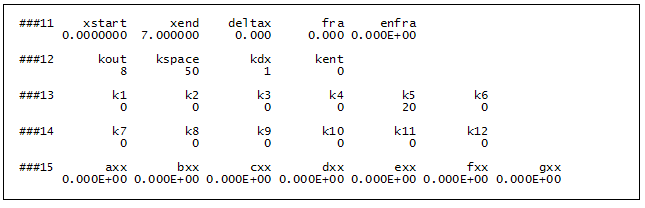### ###11

xstart  xend  deltax  fra  enfra
0.0000000  7.000000  0.000  0.000  0.000E+00

• xstart - the location for the start of computations, x = xstart

=0.0000000   in units of m. This value is consistent with the combined entry flow problem, where the flow begins at the pipe or channel entrance.

• xend - the location for the end point of computations, x = xend

=7.0000000   in units of m. The two typical choices for xend are to let xend = x(nxbc) and to let xend be defined by the problem statement, and the value of x(nxbc) can be a larger value.

• deltax - the nondimensional integration stepsize variable

=0.00    (dimensionless). When kdx is set =1, deltax is set = 0.000 and the integration stepsize is provided by the aux1(m) array.

• fra - the nondimensional variable that contributes to limiting integration stepsize

=0.000    (dimensionless). This variable is not used for internal flows (it is an entrainment variable and entrainment is never required for internal flows because the numerical domain is of fixed size).

• enfra - the nondimensional variable that contributes to limiting integration stepsize

=0.000E+00    (dimensionless). This variable is not used for internal flows (it is an entrainment variable and entrainment is never required for internal flows because the numerical domain is of fixed size).

### ###12

kout  kspace  kdx  kent
8  50  1  0

• kout - the flag to tell TEXSTAN which formatted output routine to use for the file out.txt

=8    This choice has a format designed to accompany benchmark datasets. The choice kout =4 can also print internal flows. The file out.txt is used primarily for an overview of the integration results, and it is not formatted for data analysis. The integration results are reformatted into a series of ftn.txt files for this purpose, and these files will be written when the flag k5 is set > 0.

• kspace - the flag to choose the interval for printing integration steps in the file out.txt

=50    This choice means that every 50 integration steps a line of output will be written. This flag is very useful for controlling the overall size of the file out.txt, especially for printing purposes.

• kdx - the flag to cause TEXSTAN to bypass the use of the deltax variable for integration stepsize control and instead use interpolated values from the aux1(m) array

=1    This choice means TEXSTAN will use the aux1(m) variable for integration stepsize control.

• kent - the flag for external flows onto tell TEXSTAN which dependent variables to examine for numerical accuracy related to entrainment

=0    This variable is not used for internal flows (it is an entrainment variable and entrainment is never required for internal flows because the numerical domain is of fixed size).

### ###13

k1  k2  k3  k4  k5  k6
0  0  0  0  20  0

• k1 flag is not used

• k2 flag is not used

• k3 flag is not used

• k4 flag is not used

• k5 =20 - note that when the flag is set >0 ftn.txt arrays for plotting will be printed at multiples of k5 integration steps. A description of the ftn.txt files for the internal boundary layer flow and their contents is given in the internal flows: output files section of this website.

• k6 flag is not used

### ###14

k7  k8  k9  k10  k11  k12
0  00  0  0  0  0

• k7 flag is not used

• k8 flag is not used

• k9 flag is not used

• k10 flag is not used - note that it can be reset to print velocity and temperature profiles according to the k10 definition. Because this flow is a benchmark dataset, the k10=10 option presents profiles in the nondimensional Blasius variables to examine the self-similar nature of the solution.

• k11 flag is not used

• k12 flag is not used

### ###15

axx  bxx  cxx  dxx  exx  fxx  gxx
0.000E+00  0.000E+00  0.000E+00  0.000E+00  0.000E+00  0.000E+00  0.000E+00

• axx = 0.000E+00

• bxx = 0.000E+00 - note that if k10 is set =24 (to print one set of velocity and temperature profiles), then this variable will be set according to the k10 definition.

• cxx = 0.000E+00

• dxx = 0.000E+00

• exx = 0.000E+00

• fxx = 0.000E+00

• gxx = 0.000E+00

## Data Block FOUR - Initial Conditions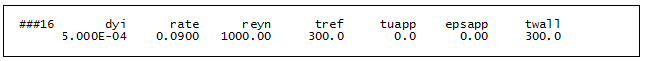### ###16

dyi  rate  reyn  tref  tuapp  epsapp  twall
5.000E-04  0.0900  1000.00  300.0  0.0  0.00  300.0

• dyi - this variable is used in the construction of the y spacing for the velocity profile, which in turn controls the grid (finite difference mesh) for TEXSTAN

=5.000E-04    This value is recommended for laminar pipe and channel flows and kstart=1. It is explained in considerable detail in the internal flows: initial profiles sections of this website.

• rate - this variable is used in the construction of the y spacing for the velocity profile, which in turn controls the grid (finite difference mesh) for TEXSTAN

=0.0900    This value is recommended for laminar pipe and channel flows and kstart=1. It is explained in considerable detail in the internal flows: initial profiles sections of this website.

• reyn - this variable is hydraulic-diameter Reynolds number for internal flows

=1000.00    This Reynolds number is specified by the problem statement.

• tref - this variable is the inlet mass-averaged (or bulk) temperature used in the formulation of the initial temperature profile for all internal geometries.

=300.0    This temperature is specified by the problem statement.

• tuapp - this variable is the inlet turbulence intensity and it used to establish the initial value of the inlet turbulence kinetic energy for one- and two-equation turbulence models

=0.00    This variable is not required because the pipe flow problem is a laminar flow. Note that it can not be left blank because it is part of the structured data input for TEXSTAN.

• epsapp - this variable establishes the inlet value of the turbulence dissipation for two-equation turbulence models

=0.00    This variable is not required because the pipe flow problem is a laminar flow. Note that it can not be left blank because it is part of the structured data input for TEXSTAN.

• twall - this variable is the inlet wall temperature used in the formulation of the initial temperature profile for all internal geometries.

=300.0    This temperature is specified by the problem statement, and it is consistent with using kstart=1 to obtain flat initial starting profiles.

website updated Sept 2010   © 1996-2010 Michael E. Crawford - all rights reserved - website validated for CSS 2.1 and XHTML 1.0 strict at www.w3.org